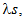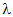# If the straight lines x = 1 + s, y = –3z = 1 +and x = t/2, y = 1 + t, z = 2 – t, with parameters s and t respectively, are co­planar, thenequals

A.
B.
C.
D.
Anonymous User Maths Two Dimensional Geometry 26 Aug, 2020 51 views